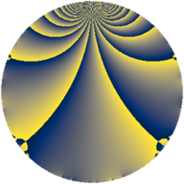# Properties

 Label 855.2.aLevel $855$ Weight $2$ Character orbit 855.a Rep. character $\chi_{855}(1,\cdot)$ Character field $\Q$ Dimension $30$ Newform subspaces $13$ Sturm bound $240$ Trace bound $11$

# Related objects

## Defining parameters

 Level: $$N$$ $$=$$ $$855 = 3^{2} \cdot 5 \cdot 19$$ Weight: $$k$$ $$=$$ $$2$$ Character orbit: $$[\chi]$$ $$=$$ 855.a (trivial) Character field: $$\Q$$ Newform subspaces: $$13$$ Sturm bound: $$240$$ Trace bound: $$11$$ Distinguishing $$T_p$$: $$2$$, $$7$$

## Dimensions

The following table gives the dimensions of various subspaces of $$M_{2}(\Gamma_0(855))$$.

Total New Old
Modular forms 128 30 98
Cusp forms 113 30 83
Eisenstein series 15 0 15

The following table gives the dimensions of the cuspidal new subspaces with specified eigenvalues for the Atkin-Lehner operators and the Fricke involution.

$$3$$$$5$$$$19$$FrickeDim.
$$+$$$$+$$$$+$$$$+$$$$3$$
$$+$$$$+$$$$-$$$$-$$$$3$$
$$+$$$$-$$$$+$$$$-$$$$3$$
$$+$$$$-$$$$-$$$$+$$$$3$$
$$-$$$$+$$$$+$$$$-$$$$6$$
$$-$$$$+$$$$-$$$$+$$$$2$$
$$-$$$$-$$$$+$$$$+$$$$3$$
$$-$$$$-$$$$-$$$$-$$$$7$$
Plus space$$+$$$$11$$
Minus space$$-$$$$19$$

## Trace form

 $$30q - 4q^{2} + 26q^{4} + 2q^{5} - 4q^{7} + O(q^{10})$$ $$30q - 4q^{2} + 26q^{4} + 2q^{5} - 4q^{7} - 8q^{11} - 4q^{13} + 12q^{14} + 10q^{16} + 4q^{17} - 2q^{20} + 16q^{22} + 12q^{23} + 30q^{25} + 28q^{26} + 12q^{28} - 4q^{29} + 8q^{31} + 16q^{32} + 24q^{34} + 4q^{35} - 8q^{37} + 6q^{38} + 4q^{41} + 4q^{43} - 24q^{44} + 4q^{46} + 4q^{47} + 14q^{49} - 4q^{50} - 28q^{52} - 16q^{53} + 8q^{55} + 44q^{56} - 24q^{58} - 32q^{59} + 4q^{61} + 8q^{62} - 38q^{64} - 4q^{67} + 68q^{68} - 20q^{70} + 4q^{73} - 4q^{74} + 24q^{77} - 16q^{79} + 6q^{80} - 16q^{82} + 28q^{83} - 20q^{85} + 20q^{86} + 44q^{88} + 12q^{89} + 16q^{91} - 44q^{92} - 4q^{94} + 8q^{95} + 16q^{97} - 52q^{98} + O(q^{100})$$

## Decomposition of $$S_{2}^{\mathrm{new}}(\Gamma_0(855))$$ into newform subspaces

Label Dim. $$A$$ Field CM Traces A-L signs $q$-expansion
$$a_2$$ $$a_3$$ $$a_5$$ $$a_7$$ 3 5 19
855.2.a.a $$1$$ $$6.827$$ $$\Q$$ None $$-1$$ $$0$$ $$-1$$ $$4$$ $$-$$ $$+$$ $$+$$ $$q-q^{2}-q^{4}-q^{5}+4q^{7}+3q^{8}+q^{10}+\cdots$$
855.2.a.b $$1$$ $$6.827$$ $$\Q$$ None $$-1$$ $$0$$ $$1$$ $$-2$$ $$-$$ $$-$$ $$+$$ $$q-q^{2}-q^{4}+q^{5}-2q^{7}+3q^{8}-q^{10}+\cdots$$
855.2.a.c $$1$$ $$6.827$$ $$\Q$$ None $$1$$ $$0$$ $$1$$ $$-2$$ $$-$$ $$-$$ $$-$$ $$q+q^{2}-q^{4}+q^{5}-2q^{7}-3q^{8}+q^{10}+\cdots$$
855.2.a.d $$2$$ $$6.827$$ $$\Q(\sqrt{2})$$ None $$-2$$ $$0$$ $$2$$ $$0$$ $$-$$ $$-$$ $$+$$ $$q+(-1+\beta )q^{2}+(1-2\beta )q^{4}+q^{5}+\beta q^{7}+\cdots$$
855.2.a.e $$2$$ $$6.827$$ $$\Q(\sqrt{2})$$ None $$-2$$ $$0$$ $$2$$ $$4$$ $$-$$ $$-$$ $$-$$ $$q+(-1+\beta )q^{2}+(1-2\beta )q^{4}+q^{5}+(2+\cdots)q^{7}+\cdots$$
855.2.a.f $$2$$ $$6.827$$ $$\Q(\sqrt{3})$$ None $$0$$ $$0$$ $$-2$$ $$-2$$ $$-$$ $$+$$ $$-$$ $$q+\beta q^{2}+q^{4}-q^{5}+(-1-\beta )q^{7}-\beta q^{8}+\cdots$$
855.2.a.g $$2$$ $$6.827$$ $$\Q(\sqrt{7})$$ None $$0$$ $$0$$ $$-2$$ $$-2$$ $$-$$ $$+$$ $$+$$ $$q+\beta q^{2}+5q^{4}-q^{5}+(-1+\beta )q^{7}+\cdots$$
855.2.a.h $$3$$ $$6.827$$ 3.3.148.1 None $$-1$$ $$0$$ $$-3$$ $$0$$ $$+$$ $$+$$ $$+$$ $$q-\beta _{1}q^{2}+(\beta _{1}+\beta _{2})q^{4}-q^{5}-\beta _{2}q^{7}+\cdots$$
855.2.a.i $$3$$ $$6.827$$ 3.3.148.1 None $$-1$$ $$0$$ $$-3$$ $$0$$ $$-$$ $$+$$ $$+$$ $$q-\beta _{1}q^{2}+(\beta _{1}+\beta _{2})q^{4}-q^{5}+2\beta _{2}q^{7}+\cdots$$
855.2.a.j $$3$$ $$6.827$$ 3.3.148.1 None $$-1$$ $$0$$ $$3$$ $$-4$$ $$+$$ $$-$$ $$-$$ $$q-\beta _{1}q^{2}+(\beta _{1}+\beta _{2})q^{4}+q^{5}+(-2+\cdots)q^{7}+\cdots$$
855.2.a.k $$3$$ $$6.827$$ 3.3.148.1 None $$1$$ $$0$$ $$-3$$ $$-4$$ $$+$$ $$+$$ $$-$$ $$q+\beta _{1}q^{2}+(\beta _{1}+\beta _{2})q^{4}-q^{5}+(-2+\cdots)q^{7}+\cdots$$
855.2.a.l $$3$$ $$6.827$$ 3.3.148.1 None $$1$$ $$0$$ $$3$$ $$0$$ $$+$$ $$-$$ $$+$$ $$q+\beta _{1}q^{2}+(\beta _{1}+\beta _{2})q^{4}+q^{5}-\beta _{2}q^{7}+\cdots$$
855.2.a.m $$4$$ $$6.827$$ 4.4.11344.1 None $$2$$ $$0$$ $$4$$ $$4$$ $$-$$ $$-$$ $$-$$ $$q-\beta _{2}q^{2}+(1+\beta _{1}-\beta _{2})q^{4}+q^{5}+(2+\cdots)q^{7}+\cdots$$

## Decomposition of $$S_{2}^{\mathrm{old}}(\Gamma_0(855))$$ into lower level spaces

$$S_{2}^{\mathrm{old}}(\Gamma_0(855)) \cong$$ $$S_{2}^{\mathrm{new}}(\Gamma_0(15))$$$$^{\oplus 4}$$$$\oplus$$$$S_{2}^{\mathrm{new}}(\Gamma_0(19))$$$$^{\oplus 6}$$$$\oplus$$$$S_{2}^{\mathrm{new}}(\Gamma_0(45))$$$$^{\oplus 2}$$$$\oplus$$$$S_{2}^{\mathrm{new}}(\Gamma_0(57))$$$$^{\oplus 4}$$$$\oplus$$$$S_{2}^{\mathrm{new}}(\Gamma_0(95))$$$$^{\oplus 3}$$$$\oplus$$$$S_{2}^{\mathrm{new}}(\Gamma_0(171))$$$$^{\oplus 2}$$$$\oplus$$$$S_{2}^{\mathrm{new}}(\Gamma_0(285))$$$$^{\oplus 2}$$